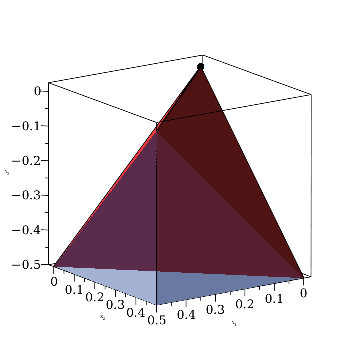ImageTools - Maple Programming Help

Home : Support : Online Help : Graphics : Image Processing : ImageTools Package : ImageTools/Scale

ImageTools

 Scale
 scale an image by the specified ratio or to the specified size

 Calling Sequence Scale( img, scale, optScaleX, opts )

Parameters

 img - Image; the image to scale scale - numeric or range(integer); scale factor or desired height range of scaled image optScaleX - (optional) numeric or range(integer); width scale factor or desired width range of image opts - (optional) equation(s) of the form option = value; specify options for the Scale command

Options

 • method = bspline or nearest
 Specifies the method of interpolation. The default method is bspline.
 • antialias = none or posint
 Specifies the size of the antialiasing filter. To skip the antialiasing, use antialias = none. The default is none.

Description

 • The Scale command rescales an image, producing a new image with the specified size.
 • The img parameter is the input image.
 • The scale parameter specifies either the desired scaling ratio, or the desired height of the scaled image. The former is expressed as a numeric value.  The latter is expressed as an integer range giving the desired resulting Array indices.
 • The optional optScaleX parameter specifies a different ratio or a desired size for the width of the resulting image. It is expressed in the same form as the scale parameter. If optScaleX is omitted, which is the usual case, the image is scaled by the same ratio in both dimensions. If scale is specified as a range, a ratio is computed from that range and that ratio is applied to the width as well as the height.
 • By default, scaling is done using bicubic B-spline interpolation, which is both reasonably fast, and produces excellent results. When reducing images using scale factors smaller than 1/2.5, the scaling is done in steps to avoid unnecessary information loss. For example, scaling by 1/4 is accomplished by scaling twice by 1/2. The nature of the algorithm makes this stepwise scaling unnecessary when enlarging an image.
 • If the method=nearest option is specified, scaling is done using the nearest-neighbor algorithm. For enlargement, this tends to produce pixelated images. For reduction, it produces grainy images. However, the algorithm is very fast and is often suitable for producing low-quality thumbnails of an image.
 • When applied to three-dimensional Arrays representing multi-channel images, for example RGB, the scaling is done separately for each channel (the channels do not interact).

Examples

 > $\mathrm{with}\left(\mathrm{ImageTools}\right):$
 > $\mathrm{img}≔\mathrm{Create}\left(200,300\right):$
 > $\mathbf{for}\phantom{\rule[-0.0ex]{0.3em}{0.0ex}}i\phantom{\rule[-0.0ex]{0.3em}{0.0ex}}\mathbf{to}\phantom{\rule[-0.0ex]{0.3em}{0.0ex}}300\phantom{\rule[-0.0ex]{0.3em}{0.0ex}}\mathbf{do}\phantom{\rule[-0.0ex]{0.0em}{0.0ex}}\phantom{\rule[-0.0ex]{2.0em}{0.0ex}}\mathrm{img}\left[1..200,i\right]≔\mathrm{evalf}\left(0.5+0.5\mathrm{sin}\left(\left(0.005+0.003i\right)i\right)\right)\phantom{\rule[-0.0ex]{0.0em}{0.0ex}}\mathbf{end}\phantom{\rule[-0.0ex]{0.3em}{0.0ex}}\mathbf{do}:$
 > $\mathrm{Embed}\left(\mathrm{img}\right)$> $\mathrm{img_near}≔\mathrm{Scale}\left(\mathrm{img},0.35,\mathrm{method}=\mathrm{nearest},\mathrm{antialias}=\mathrm{none}\right):$
 > $\mathrm{img_bspl}≔\mathrm{Scale}\left(\mathrm{img},0.35,\mathrm{antialias}=\mathrm{none}\right):$
 > $\mathrm{img_near_aa}≔\mathrm{Scale}\left(\mathrm{img},0.35,\mathrm{method}=\mathrm{nearest}\right):$
 > $\mathrm{img_bspl_aa}≔\mathrm{Scale}\left(\mathrm{img},0.35\right):$
 > $\mathrm{Embed}\left(\left[\left[\mathrm{img_near},\mathrm{img_bspl}\right],\left[\mathrm{img_near_aa},\mathrm{img_bspl_aa}\right]\right]\right)$> $\mathrm{img_near2}≔\mathrm{Scale}\left(\mathrm{img_near},\frac{1}{0.35},\mathrm{method}=\mathrm{nearest}\right):$
 > $\mathrm{img_bspl2}≔\mathrm{Scale}\left(\mathrm{img_bspl},\frac{1}{0.35}\right):$
 > $\mathrm{img_near_aa2}≔\mathrm{Scale}\left(\mathrm{img_near_aa},\frac{1}{0.35},\mathrm{method}=\mathrm{nearest}\right):$
 > $\mathrm{img_bspl_aa2}≔\mathrm{Scale}\left(\mathrm{img_bspl_aa},\frac{1}{0.35}\right):$
 > $\mathrm{Embed}\left(\left[\left[\mathrm{img_near2},\mathrm{img_bspl2}\right],\left[\mathrm{img_near_aa2},\mathrm{img_bspl_aa2}\right]\right]\right)$>